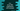# C++ array::fill() STL, standard template library explanation with example## C++ array::fill() STL, standard template library explanation with example:

array::fill() is a part of C++ standard template library or STL. This method is used to set one value to all members of an array. This function is useful if you want to fill all members of the array with a specific value.

We need to set the same type of value for an array. For an integer array, we need to fill with an integer and for a string array, we need to pass one string. For any different value type, type casting is done.

In this post, we will learn how array::fill() works with examples.

### Syntax of array::fill():

The syntax of array::fill() is as below:

``void fill(const value_type& val);``

Here, value_type is the type of value and val is the value to fill the array with. It returns none.

### Example of array::fill():

Let’s take a look at the below program:

``````#include <iostream>
#include <array>

using namespace std;

int main()
{
array<int, 5> inputArray;

inputArray.fill(-1);

cout << "Array values : " << endl;
for (int item : inputArray)
{
cout << item << ' ';
}
}``````

It will print the below output:

``````Array values :
-1 -1 -1 -1 -1``````

As you can see that all items are changed to -1.

### array::fill() with string array:

It works in same way for a string array:

``````#include <iostream>
#include <array>

using namespace std;

int main()
{
array<string, 5> stringArray;

stringArray.fill("hello");

cout << "Array values : " << endl;
for (string item : stringArray)
{
cout << item << ' ';
}
}``````

It will print:

``````Array values :
hello hello hello hello hello``````